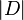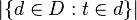## Tuesday, September 24, 2013

### Implementation of TF/IDF (Term Frequency-Inverse Document Frequency) in JAVA

Tf–Idf is the product of two statistics, term frequency and inverse document frequency. Various ways for determining the exact values of both statistics exist. In the case of the term frequency tf(t,d), the simplest choice is to use the raw frequency of a term in a document, i.e. the number of times that term t occurs in document d. If we denote the raw frequency of t by f(t,d), then the simple Tf scheme is tf(t,d) = f(t,d).

The inverse document frequency is a measure of whether the term is common or rare across all documents. It is obtained by dividing the total number of documents by the number of documents containing the term, and then taking the logarithm of that quotient .

Where:$|D|$  is the cardinality, or the total number of documents in the corpus and respectively$|\{d \in D: t \in d\}|$ is the number of documents where the term appears.

Then tf–idf is calculated as:

A high weight in tf–idf is reached by a high term frequency (in the given document) and a low document frequency of the term in the whole collection of documents; the weights hence tend to filter out common terms. Since the ratio inside the idf's log function is always greater than or equal to 1, the value of idf (and tf-idf) is greater than or equal to 0. As a term appears in more documents, the ratio inside the logarithm approaches 1, bringing the idf and tf-idf closer to 0.

NOTE: For a deeper dive into theory read this.

Practically..

A possible implementation (written in Java) for calculating Tf/Idf of the terms of a document is given below:

public class Tf_Idf
{
/**
* Calculated the tf of term termToCheck
* @param totalterms : Array of all the words under processing document
* @param termToCheck : term of which tf is to be calculated.
* @return tf(term frequency) of term termToCheck
*/
public double tfCalculator(List totalterms, String termToCheck)
{
double count = 0;
for (String s : totalterms)
{
if (s.equalsIgnoreCase(termToCheck))
count++;
}
return count / totalterms.length;
}

/**
* Calculated idf of term termToCheck
* @param allTerms : all the terms of all the documents
* @param termToCheck
* @return idf(inverse document frequency) score
*/
public double idfCalculator(List<> allTerms, String termToCheck)
{
double count = 0;
for (String[] ss : allTerms)
{
for (String s : ss)
{
if (s.equalsIgnoreCase(termToCheck))
{
count++;
break;
}
}
}
return Math.log(allTerms.size() / count);
}

}

*If you want to know more about tf/idf and its applications check out the Cosine similarity example here!